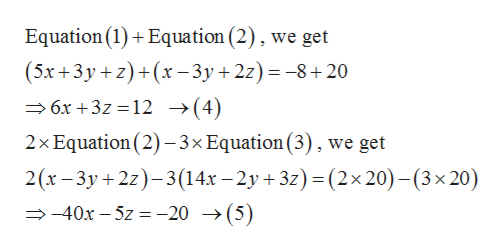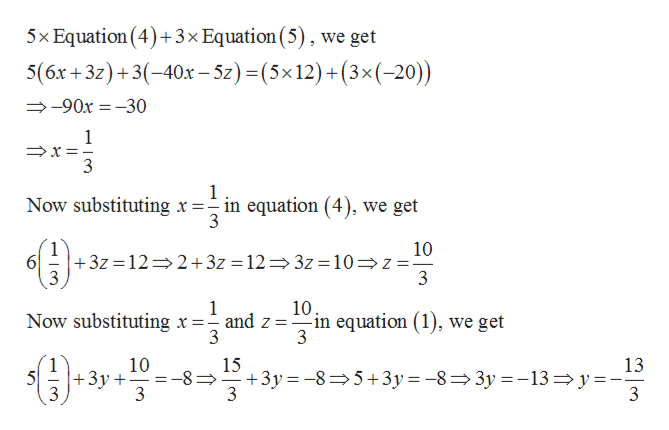# I am stuck on this equation and unsure how to solve. Here is the equationSolve by system of equations:  5x+3y+z=−8x−3y+2z=2014x−2y+3z=20

Question

I am stuck on this equation and unsure how to solve. Here is the equation

Solve by system of equations:

 5x+3y+z = −8 x−3y+2z = 20 14x−2y+3z = 20

check_circleExpert Solution
Step 1

Given,

Step 2help_outlineImage TranscriptioncloseEquation (1) Equation (2), we get (5x+3y z)+(x-3y +2z)8+20 6x+3z 12 -»(4) 2x Equation (2)-3x Equation (3), we get 2(x-3y 2z-3(14x -2y +3z) (2x 20) -(3 x 20) 40x-5z= -20 ->(5) fullscreen
Step 3

Now solving equations...help_outlineImage Transcriptionclose5x Equation (4)+3x Equation (5), we get 5(6x+3z)+3(-40x - 5z) = (5x12)+(3x(-20)) -90x-30 1 3 1 Now substituting x=-in equation (4), we get 3 1 +3z 12 2+3z = 12 3z =10z 10 61 10 Now substituting x =-and z =in equation (1), we get 3 3 10 +3y+ 3 15 +3y 85+3y -8= 3y13 y- 3 13 5 _ 3 fullscreen

### Want to see the full answer?

See Solution

#### Want to see this answer and more?

Solutions are written by subject experts who are available 24/7. Questions are typically answered within 1 hour*

See Solution
*Response times may vary by subject and question
Tagged in

### Algebra